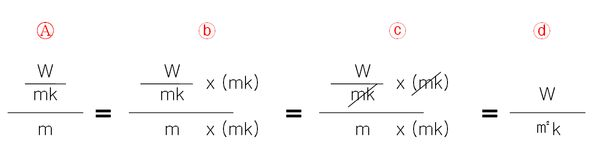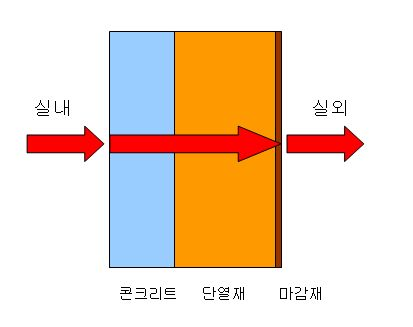기술자료E.2-01. A Word About Thermal Conductivity, Thermal Transmittance and Thermal Resistance

M 관리자 0 306 2018.12.20 22:04

2013.05.20: Regional Thermal Transmittance Regulation Table handled as a separate link (to ease updates)

2012.01.06: Additional Data on Thermal Conductivity and Moisture Resistance Coefficients

2010.04.06: Additional Data on Thermal Resistance Performance of Cavity Walls

-------------------------------------------------

Many terms and definitions must be known when speaking about passive house; however, when speaking about insulation, the three terms that must be understood are thermal conductivity, thermal transmittance and thermal resistance.

Let us pass on the definitions for now. The Internet is overflowing with definitions for these three terms. Of course, there are a lot of rogue websites with inaccurate data; nevertheless, we will pass.

The dictionary definition of Thermal Conductivity can be found at:

The dictionary definition of Thermal Transmittance can be found at:

In this article, unless otherwise specified, all calculations regarding insulation are in reference to EPS Type 1 (thermal conductivity: 0.034 W/mk). And in this section, you will find some explanations that are not based on purely scientific methods. Rather, precedence was given to ease of comprehension, so your understanding is requested.

PHIKO, whenever possible, has unified all units of thermal energy into Watts (W).

(Note: 1 W/mK = 0.86 kcal/mh℃)

A. What is Thermal Conductivity?

It is the rate at which heat passes through 1m thickness of a material expressed in W/mK or kcal/mh℃.

If the thickness of 1m of material is decreased or increased, the thermal property is expressed in terms of Thermal Transmittance. So, Thermal Conductivity is a thermal property with respect to a unit thickness of 1m.

In other words, if all the materials in the world were 1m in thickness, then the rate of heat transfer would be expressed solely in terms of Thermal Conductivity.

If the thickness changes, the Thermal Conductivity is divided by the Thickness to get the Thermal Transmittance.

That is, Thermal Conductivity ÷ Thickness = Thermal Transmittance; and the lower the number, the higher the performance.

Thermal Conductivity / Thickness (m) = Thermal Transmittance

B. What is Thermal Transmittance (U-Value)?

The thermal conductivity of a material with a certain thickness is expressed as its U-value and the unit of expression is W/㎡K.

The calculation method, as mentioned before, is Thermal Conductivity ÷ Thickness; and it should be noted that the thickness is expressed in meters.

If you try to understand the concept with the unit in mind, it becomes easier to memorize the unit and facilitates overall comprehension.A. Thermal Transmittance is Thermal Conductivity divided by Thickness, so W/mK ÷ m

b. Multiply (mk) with the numerator and denominator

c. Simplify the fraction

d. The resulting unit is W/㎡k

For example, if we input EPS Type 1 into the equation, it has a thickness of 10cm so the Thermal Transmittance (U-value) of the insulation would be:

0.034 W/mk ÷ 0.1 m = 0.34 W/㎡k

Here again, the lower the number, the better the performance.

If a material has a thickness of 1m, then Thermal Transmittance = Thermal Conductivity

Then how are composite materials calculated? To answer this question, we must understand Thermal Resistance.

C. What is Thermal Resistance (R-Value)?

Thermal Resistance is 1 ÷ Thermal Transmittance. That is, the reciprocal of Thermal Transmittance. Therefore, the calculation is Thickness ÷ Thermal Conductivity. The unit of measure is ㎡K/W, and is also called the R-Value

Since Thermal Resistance (R-value) is the reciprocal of the Thermal Transmittance (U- value), the higher the number, the better the performance.

The Thermal Transmittance of composite materials is not the sum of the thermal transmittance of each of the separate components. (VERY IMPORTANT)

First, find the sum of the Thermal Resistances and then find the reciprocal.

Thickness (m)  / Thermal Conductivity = Thermal Resistance

For example, for a wall composed of 20cm concrete (1.73 W/mK) and 10cm EPS Type 1 Insulation (0.034 W/mK), the Thermal Transmittance would be found as follows:

a. Thermal Resistance of Concrete = 0.2/1.73 = 0.116

b. Thermal Resistance of Insulation = 0.1/0.034 = 2.94

c. Total Thermal Resistance = 0.116 + 2.94 = 3.056 ㎡K/W

d. Total Thermal Transmittance = 1/3.056 = 0.327 W/㎡K

D. Passive House Exterior Wall Calculation Example

According to PHI, the exterior wall assembly of a passive house should have a thermal transmittance of less than 0.15 W/㎡K.

To achieve this value with insulation alone, how thick must the EPS Type 1 Insulation be?

Since

Thermal Conductivity ÷ Thickness = Thermal Transmittance,

Thermal Conductivity ÷ Thermal Transmittance = Thickness

Therefore, for the Thermal Transmittance of the exterior wall to be 0.15 W/㎡K,

0.034 ÷ 0.15 = 0.226m; so, 226mm EPS Type 1 Insulation must be installed in order to satisfy this requirement.

With the exception of insulation, the thermal conductivity of materials such as concrete is high, so they do not contribute much to improving the thermal transmittance value of the wall assembly. Therefore the quickest way to approximate the thermal transmittance of the wall assembly in the planning stages is to just calculate for varying thicknesses of insulation.

E. Surface Thermal Transfer Resistance, the last thing to be aware of.

The diagram below, showing the sequence of thermal transfer in a wall assembly, is a visual aid to ease comprehension of how surface transfer resistance works.

InsideOutside

Concrete /  Insulation / Outside Finish Material

That is, the heat in the room must (1) penetrate the wall; (2) pass through the wall; and (3) penetrate the wall again and exit. (Winter Climate)

Resistance occurs in 1 and 3.

1 is the inside surface thermal transfer resistance and 3, the outside surface thermal transfer resistance. In other words, there is a force to resist the transfer of heat through the wall assembly in the beginning and in the end of the wall assembly.

This is because the air near the surface of an object acts as an element of thermal resistance. (This is a simplified juxtaposition of convective heat resistance and radiant heat resistance to avoid an exhaustive explanation).

For Surface Thermal Transfer Resistance values, please refer to the "Energy Conservation Design Guidelines for Buildings." Actually, just reading through the "Energy Conservation Design Guidelines for Buildings" will facilitate comprehension of how buildings use energy. (It is a testimony to how well made Korea's laws are.)

Therefore, when calculating the thermal transmittance of a wall assembly, it is necessary to input the inside (Ri) and outside (Ro) surface thermal transfer resistance values. (If these values are not inputted in the energy analysis program, the calculation will be incorrect.)

An excerpt of the rules for outside walls follows:

** 표면열전달저항 [단위:㎡·K/W]

 Thermal Resistance    Building Component Interior Surface Thermal Transfer Resistance, Ri[Unit: ㎡·K/W] Outside Surface Thermal Transfer Resistance, Ro [Unit: ㎡·K/W] Indirect Contact with Outside Air Direct Contact with Outside Air Outside Wall of Living Room (Includes Party Walls, Windows and Doors) 0.11 0.11 0.043 Living Room Floor of Lowest Level 0.086 0.15 0.043 Ceiling or Roof of Highest Level Living Room 0.086 0.086 0.043 Floor between Apt. Levels 0.086(0.10) - -

※ [Attached Table 5] Interior and Outside Surface Thermal Transfer Resistance Values for Thermal Transmittance Calculation.

Simply put, it is much easier for heat to escape through the wall from the inside to the outside than the reverse, to enter from the outside to the inside through the wall.

Therefore, if we plug in the numbers into the Equation in "C" we get the proper long version as follows:

Inside Surface Thermal Transfer Resistance: Ri = 0.11 ㎡K/W

a Thermal Resistance of Concrete = 0.2/1.73 = 0.116

b Thermal Resistance of Insulation = 0.1/0.034 = 2.94

Outside Surface Thermal Transfer Resistance: Ro = 0.043 ㎡K/W

c Total Thermal Resistance = 0.11 + 0.116 + 2.94 + 0.043 = 3.209 ㎡K/W

d Total Thermal Transmittance = 1/3.209 = 0.312 W/㎡K

I can hear voices asking, why bother with the long version when there is not much difference? Hmmm… just saying that this is the proper process is all.

** To give a more direct example, we can compare thermal conductivity to the fuel efficiency of a car. For example, if a car goes 15km on 1L, let us substitute thermal conductivity for fuel efficiency. If we fill the tank with 20L of fuel, how many km can the car go? 15 x 20 = 300km. This 300km then is the thermal transmittance, and when we invert the value, 1/300 = 0.003 we get the car's "distance resistance," so to speak. This is the same concept as thermal resistance.

A more detailed explanation of surface thermal transfer resistance can be found in:

For reference, let us take a look at the thermal resistance in cavity walls

[Standard Attached Table 5]

Thermal Resistance of Cavity Wall Values in Thermal Transmittance Calculations

Types of Air Spaces
Air Space Thickness da (cm)

Air Space Thermal Resistance

Ra [SI Unit: ㎡K/W]

(1) Factory-Manufactured Airtight Product
Less than 2 cm
0.086×da(cm)
More than 2 cm
0.17
(2) On-Site Construction, etc.
Less than 1 cm
0.086×da(cm)
More than 1 cm
0.086
(3) When the emissivity of the air space is less than 0.5 with reflective insulation
1.5 times (1) or (2)

The thermal conductivity of air is very good, as is well known. At (0.025W/mk), it is even better than many types of insulation.

People have asked, then at times, can the air space layer which is sandwiched between the exterior finish material and the insulation layer, serve as insulation? Simply put, NO, because air is a fluid.

For air to have insulating properties it must basically be sealed. Since a building cannot be completely sealed, save for perhaps the air inside double paned windows and the like, we can conclude that air cannot have insulating properties. If we refer to the Standard Attached Table above, for On-Site Construction, where the air space is more than 1cm, the thermal resistance (R) value is 0.086 ㎡k/W, regardless of thickness. When converted to its thermal transmittance (U) value, it is 11.62 W/㎡k, a value that can be disregarded as having any significant insulating properties.

Regional Thermal Transmittance Limits can be found in the following link:

http://www.phiko.kr/bbs/board.php?bo_table=z3_01&wr_id=155

** Up to this point we have covered the most difficult portion of Energy Analysis. For architectural designers, it has probably been especially painful. However it is a hurdle that must be overcome. If you reread the section a few times, I believe it will become clear.

If anyone has found an easier explanation of Thermal Conduction, Transmittance and Resistance anywhere, please do not hesitate to make recommendations. The Pritzker Prize, essentially the Nobel Prize in Architecture, should be awarded to this person.

PHIKO's Thermal Transmittance Program

** For Reference, a Table of Thermal Conduction Values for Building Materials

* Thermal Conduction of Insulation

The above thermal conduction values are general knowledge; however, the precise conductivity values should be confirmed with the product supplier's test result report.

* Thermal Conduction of Other Building Materials

** Note

KS: Korea Energy Conservation Design Guidelines for Buildings

DIN: German Institute for Standardization

ETC: Miscellaneous References

* Standard values other than those designated by KS are not recognized in the thermal transmittance calculations of Korea's Energy Performance Index (EPI)

The above thermal conduction values are general knowledge; however, the precise conductivity values should be confirmed with the product supplier's test result report.